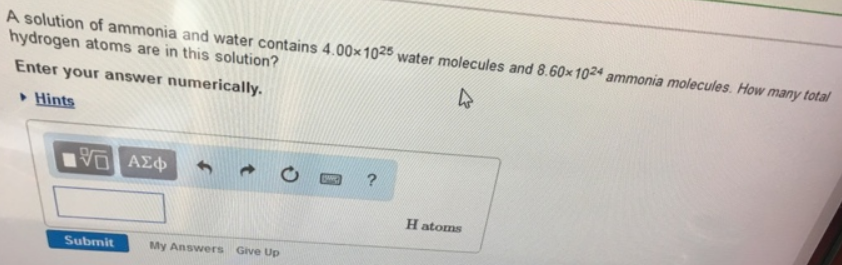# Problem: A solution of ammonia and water contains 4.00 x 1025 water molecules and 8.60 x 1024 ammonia molecules How many total hydrogen atoms are in this solution? Enter your answer numerically.

###### FREE Expert Solution

Molecular formula of ammonia is NH3 and water is H2O. There are 3 Hs in NH3 and 2 in H2O, therefore the total amount of Hs are:

91% (39 ratings)###### Problem Details

A solution of ammonia and water contains 4.00 x 1025 water molecules and 8.60 x 1024 ammonia molecules How many total hydrogen atoms are in this solution?